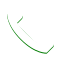# What even is dB FS?

What really are Decibels?
Decibels simply put are a way to take large values of measurements and convert them into a simpler more understandable set of numbers. Decibel is 1/10th of a Bel, named after Alexander Graham Bell who first used them to measure the loss of signals over telephone lines.

Decibels come in many different forms, as we commonly used to understand, decibels are not really a unit of measurement in themselves.

This is the simple formula to calculate any ratio as a decibel. Pay attention to the ratio aspect because a decibel really is always a measurement of a ratio. Without a ratio you cannot have a decibel value.

Imagine this,you have two very wide ranges of numbers, let’s say 1 and 16777216 to measure the value of the loudness of a sound. Would this be an easy or convenient way to measure the loudness?
If the sound was very loud you would have to say that the value of this sound is lets say somewhere close to 1500081 or if it’s soft it would be somewhere at 1000. This is an extremely inconvenient way to measure loudness or represent it in terms of numbers.
This is where decibels step in, if you plug the two values 1 and 16777216 into the above equation you will find

## dB = 20 * log (16777216/1) = 144 dB

This is exactly how we arrive at the calculation for the Dynamic Range of a 24bit
A 24 bit system means that a system that is capable of measuring up 2 to the power 24 values.
2^24= 16777216 which are the maximum possible values that the 24 bit system can hold.

In a Digital system with is 24bit, the least possible value that we can hold is 1 whereas the maximum possible value that we can hold is 16777216, so when converted to dB this gives us a value of 144dB to 0 dB
This is what the meter in your DAW represents, infact its inverted in a DAW from -144 to 0dB where 0dB is the absolute value above which your digital system cannot read information which in turn leads to what we call as clipping.

## Dynamic Range:

Dynamic range is the measurement of range between the highest and the lowest value that a system can measure. Say if the softest sound was at -40dB and the loudest sound was at 0dB, what would the dynamic range be?
40dB
The same way in a digital system with a bit depth of 24 the dynamic range is -144dB to 0dB
DR of a 24 bit system is 144dB FS

The same way for a 16 bit system the DR would be 96dB FS

This measurement of dB of the total DR of a digital system is what we denote as dB FS

## Get in touch!

If you guys have another other questions you would like to shoot at me, just shoot me a mail at ronak@gray-spark.com.
ExclusiveCall Now